﻿ MechDesigner Reference & User Interface > Dialog-Boxes > Dialog: Function-Block: Gearing

# Dialog: Function-Block: Gearing

Navigation:  MechDesigner Reference & User Interface > Dialog-Boxes >

# Dialog: Function-Block: Gearing

## Gearing FB

Use Gearing FB to transform motion-values with a linear mathematical function.

Default Output = Input

### Open Gearing FB dialog-box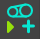Edit the Gearing FB

 1.Double-click the Gearing FB in the graphic-area. - or - 1.

The Gearing FB dialog-box is now open.

Gearing FB dialog-box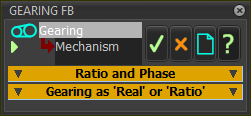The Gearing FB function is: Output  = {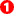× (Input +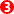) } +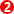Ratio and Phase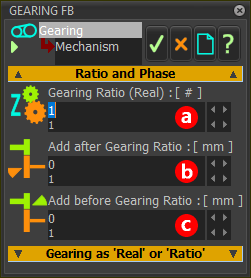Gearing FB dialog-box

Edit one to three ParametersGearing Ratio (See also below Gearing as 'Real' or 'Ratio' )Add after Gearing-RatioAdd before Gearing-Ratio

Example:

 Gearing-Ratio =1.5 Add After Gearing-Ratio = 83 Add Before Gearing-Ratio = 23 Input = 37 Output = (1.5*(37+23))+83 = 173

Gearing as 'Real' or 'Ratio'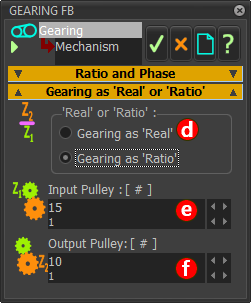Gearing FB dialog-box

You can enter the Gearing Ratio in two ways: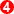Gearing as 'Real'   Enter a real number in the Gearing Ratio- see aboveGearing as 'Ratio' Enter two integers in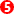&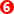Gearing Ratioas RatioInput-Pulley = ± All Integers, including zeroOutput-Pulley = Positive Integer ≥ 1